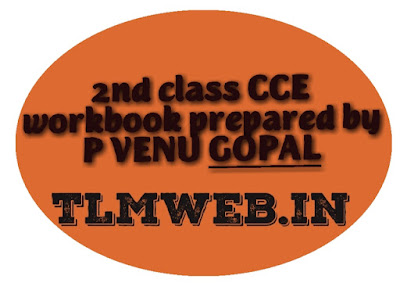Breaking News:

More ...

More ...

More ...

More ...

More ...

More ...

More ...

## Friday, June 14, 2019## TLMweb® 2nd class Maths  CCE workbook2nd class Maths CCE work book Prepared by P. VENU GOPAL M.P.P SCHOOL P. Kothapalli BUKKARAYA SAMUDRAM md, ANANATHAPURAM DT

Workbooks are often used in schools for younger students, either in middle school or elementary school. They are favored because students can work directly in their books, eliminating the need for looseleaf and copying questions from a textbook. In industry, they may be customized interactive manuals which are used to help provide structure to an otherwise complex problem.
Workbook usually covers important concepts and tasks related to syllabus. Workbooks are used for solving extra problems and concepts which students have already studied from textbook.
Workbooks also hold an advantage because they are usually smaller and lighter than textbooks, which equates to less trouble when the student brings the book home to complete their homework.
The term workbook is also used to describe other compilations of questions that require the reader to complete scratch-work when dealing with higher-level mathematics. It can also be used as a training tool for certain job positions.

Maths work sheets, Maths work books,ap 2nd class Chapter wise Maths work sheets2ndclass Maths work book-Abhyaasa deepika, 2nd class Maths work book, Maths books pdf, Maths work sheets, Maths worksheets, Maths basics, ap&nbsp; Maths textbooks pdf,2nd class Maths worksheets, 2nd class Maths work sheets, Maths work books,ap 2nd class Chapter wise Maths work sheets Maths work sheets, Maths work books,ap 2nd class Chapter wise Maths work sheets Maths work sheets, Maths work books,ap 2nd class Chapter wise Maths work sheets2ndclass Maths work book-Abhyaasa deepika, 2nd class Maths work book, Maths books pdf, Maths work sheets, Maths worksheets, Maths basics, ap&nbsp; Maths textbooks pdf,2nd class Maths worksheets, 2nd class Maths work sheets, Maths work books,ap 2nd class Chapter wise Maths work sheets Maths work sheets, Maths work books,ap 2nd class Chapter wise Maths work sheets Maths work book-Abhyaasa deepikav

Thanks for reading TLMweb® 2nd class Maths CCE workbook